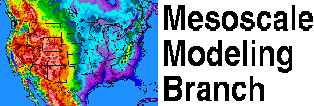Long Term Comparisons of the Eta/NAM vs. the MRF/GFS

This page shows some comparisons of the verification numbers between the NAM (formerly the Eta [1993-2006], then the WRF-NMM [2006-2011], now the NEMS-NMMB [2011-present]) model and the GFS (formerly MRF). These statistics start from 1993, the earliest statistics are available for the Eta/NAM. Presented are quarterly 12-month running means.

Variable and Level Jan-Dec Apr-Mar Jul-Jun Oct-Sep
850 mb Z X X X X
500 mb Z X X X X
250 mb Z X X X X
850 mb T X X X X
500 mb T X X X X
250 mb T X X X X
850 mb VWND X X X X
500 mb VWND X X X X
250 mb VWND X X X X

The statistics in the chart below show comparisons between the AVN and the MRF. Both these models are the basis of the GFS. These statistics start in 1985. Presented are quarterly 12-month running means.

Variable and Level Jan-Dec Apr-Mar Jul-Jun Oct-Sep
850 mb Z X X X X
500 mb Z X X X X
250 mb Z X X X X
850 mb T X X X X
500 mb T X X X X
250 mb T X X X X
850 mb VWND X X X X
500 mb VWND X X X X
250 mb VWND X X X X

DISCLAIMER

PRIVACY / COLLECTION OF INFORMATION STATEMENT